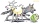# Triangular prism

Calculate the surface of a triangular prism 10 cm high, the base of which is a triangle with sides 6 cm 8 cm and 8 cm

Result

S =  264.497 cm2

#### Solution:Try calculation via our triangle calculator.

Leave us a comment of example and its solution (i.e. if it is still somewhat unclear...):

Showing 0 comments:Be the first to comment!#### To solve this example are needed these knowledge from mathematics:

See also our trigonometric triangle calculator.

## Next similar examples:

1. Regular triangular prismCalculate the surface area of body of regular triangular prism, when the length of its base edge is 6.5 cm and height 0.2 m.
2. Triangular prismCalculate the volume and surface of the triangular prism ABCDEF with base of a isosceles triangle. Base's height is 16 cm, leg 10 cm, base height vc = 6 cm. The prism height is 9 cm.
3. Triangular prismCalculate the surface of a regular triangular prism with a bottom edge 8 of a length of 5 meters and an appropriate height of 60 meters and prism height is 1 whole 4 meters.
4. The farmerThe farmer would like to first seed his small field. The required amount depends on the seed area. Field has a triangular shape. The farmer had fenced field, so he knows the lengths of the sides: 119, 111 and 90 meters. Find a suitable way to determine th
5. Surface area 6Find the surface area of a prism whose bases are right triangles with sides of length 3, 4, and 5 inches and a height of 8 inches. Include a sketch
6. BasenHow many square meters of tiles we need to tile the walls and floor of the pool 15 meters long, six meters wide and two meters?deep
7. Pool tilesThe pool is 25m long, 10m wide and 160cm deep. How many m2 of tiles will be needed on the walls and the pool? How many pieces of tile are needed when 1 tile has a square shape with a 20cm side? How much does it cost when 1m2 of tiles costs 258 Kc?
8. TriangleThe triangle has known all three sides: a=5.5 m, b=5.3 m, c= 7.8 m. Calculate area of ​this triangle.
9. MedianThe median of the triangle LMN is away from vertex N 84 cm. Calculate the length of the median, which start at N.
10. DiamondRhombus has side 17 cm and and one of diagonal 22 cm long. Calculate its area.
11. TriangleCalculate heights of the triangle ABC if sides of the triangle are a=75, b=84 and c=33.
12. Area of a triangleWhat is the area of a triangle that has the base 4 1/4 and the height of 3 3/3?
13. An equilateralAn equilateral triangle with a side 10 m represents a wooden platform standing in a lawn. A goat is tied to a corner with a 15 m rope. What is the maximum amount of grazing area available to the goat?
14. Center traverseIt is true that the middle traverse bisects the triangle?
15. One trapeziumOne trapezium has AB=24M, BC=36M, CD=80M, DA=80M long sides. Find the area.
16. TriangleProve whether you can construct a triangle ABC, if a=9 cm, b=10 cm, c=4 cm.
17. Is right triangle or notIf right triangle ABC, have sides a=13, b=11.5, c=22.5. Find area.